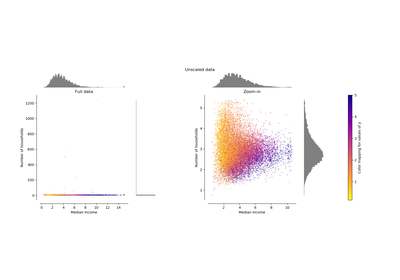# `sklearn.preprocessing`.QuantileTransformer¶

class `sklearn.preprocessing.``QuantileTransformer`(n_quantiles=1000, output_distribution=’uniform’, ignore_implicit_zeros=False, subsample=100000, random_state=None, copy=True)[source]

Transform features using quantiles information.

This method transforms the features to follow a uniform or a normal distribution. Therefore, for a given feature, this transformation tends to spread out the most frequent values. It also reduces the impact of (marginal) outliers: this is therefore a robust preprocessing scheme.

The transformation is applied on each feature independently. The cumulative density function of a feature is used to project the original values. Features values of new/unseen data that fall below or above the fitted range will be mapped to the bounds of the output distribution. Note that this transform is non-linear. It may distort linear correlations between variables measured at the same scale but renders variables measured at different scales more directly comparable.

Read more in the User Guide.

Parameters: n_quantiles : int, optional (default=1000) Number of quantiles to be computed. It corresponds to the number of landmarks used to discretize the cumulative density function. output_distribution : str, optional (default=’uniform’) Marginal distribution for the transformed data. The choices are ‘uniform’ (default) or ‘normal’. ignore_implicit_zeros : bool, optional (default=False) Only applies to sparse matrices. If True, the sparse entries of the matrix are discarded to compute the quantile statistics. If False, these entries are treated as zeros. subsample : int, optional (default=1e5) Maximum number of samples used to estimate the quantiles for computational efficiency. Note that the subsampling procedure may differ for value-identical sparse and dense matrices. random_state : int, RandomState instance or None, optional (default=None) If int, random_state is the seed used by the random number generator; If RandomState instance, random_state is the random number generator; If None, the random number generator is the RandomState instance used by np.random. Note that this is used by subsampling and smoothing noise. copy : boolean, optional, (default=True) Set to False to perform inplace transformation and avoid a copy (if the input is already a numpy array). quantiles_ : ndarray, shape (n_quantiles, n_features) The values corresponding the quantiles of reference. references_ : ndarray, shape(n_quantiles, ) Quantiles of references.

`quantile_transform`
Equivalent function without the estimator API.
`StandardScaler`
perform standardization that is faster, but less robust to outliers.
`RobustScaler`
perform robust standardization that removes the influence of outliers but does not put outliers and inliers on the same scale.

Notes

For a comparison of the different scalers, transformers, and normalizers, see examples/preprocessing/plot_all_scaling.py.

Examples

```>>> import numpy as np
>>> from sklearn.preprocessing import QuantileTransformer
>>> rng = np.random.RandomState(0)
>>> X = np.sort(rng.normal(loc=0.5, scale=0.25, size=(25, 1)), axis=0)
>>> qt = QuantileTransformer(n_quantiles=10, random_state=0)
>>> qt.fit_transform(X)
array([...])
```

Methods

 `fit`(X[, y]) Compute the quantiles used for transforming. `fit_transform`(X[, y]) Fit to data, then transform it. `get_params`([deep]) Get parameters for this estimator. `inverse_transform`(X) Back-projection to the original space. `set_params`(**params) Set the parameters of this estimator. `transform`(X) Feature-wise transformation of the data.
`__init__`(n_quantiles=1000, output_distribution=’uniform’, ignore_implicit_zeros=False, subsample=100000, random_state=None, copy=True)[source]
`fit`(X, y=None)[source]

Compute the quantiles used for transforming.

Parameters: X : ndarray or sparse matrix, shape (n_samples, n_features) The data used to scale along the features axis. If a sparse matrix is provided, it will be converted into a sparse `csc_matrix`. Additionally, the sparse matrix needs to be nonnegative if ignore_implicit_zeros is False. self : object Returns self
`fit_transform`(X, y=None, **fit_params)[source]

Fit to data, then transform it.

Fits transformer to X and y with optional parameters fit_params and returns a transformed version of X.

Parameters: X : numpy array of shape [n_samples, n_features] Training set. y : numpy array of shape [n_samples] Target values. X_new : numpy array of shape [n_samples, n_features_new] Transformed array.
`get_params`(deep=True)[source]

Get parameters for this estimator.

Parameters: deep : boolean, optional If True, will return the parameters for this estimator and contained subobjects that are estimators. params : mapping of string to any Parameter names mapped to their values.
`inverse_transform`(X)[source]

Back-projection to the original space.

Parameters: X : ndarray or sparse matrix, shape (n_samples, n_features) The data used to scale along the features axis. If a sparse matrix is provided, it will be converted into a sparse `csc_matrix`. Additionally, the sparse matrix needs to be nonnegative if ignore_implicit_zeros is False. Xt : ndarray or sparse matrix, shape (n_samples, n_features) The projected data.
`set_params`(**params)[source]

Set the parameters of this estimator.

The method works on simple estimators as well as on nested objects (such as pipelines). The latter have parameters of the form `<component>__<parameter>` so that it’s possible to update each component of a nested object.

Returns: self :
`transform`(X)[source]

Feature-wise transformation of the data.

Parameters: X : ndarray or sparse matrix, shape (n_samples, n_features) The data used to scale along the features axis. If a sparse matrix is provided, it will be converted into a sparse `csc_matrix`. Additionally, the sparse matrix needs to be nonnegative if ignore_implicit_zeros is False. Xt : ndarray or sparse matrix, shape (n_samples, n_features) The projected data.

## Examples using `sklearn.preprocessing.QuantileTransformer`¶Compare the effect of different scalers on data with outliers